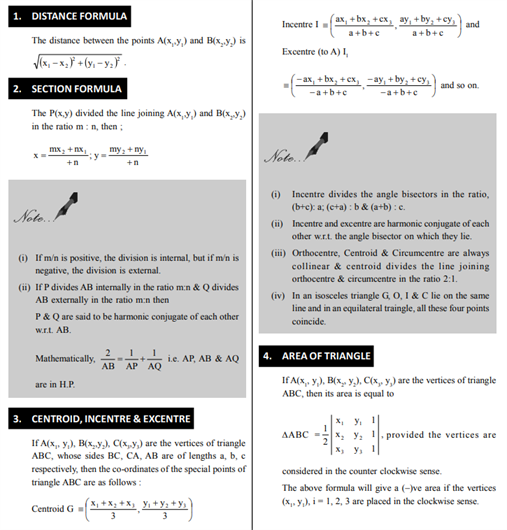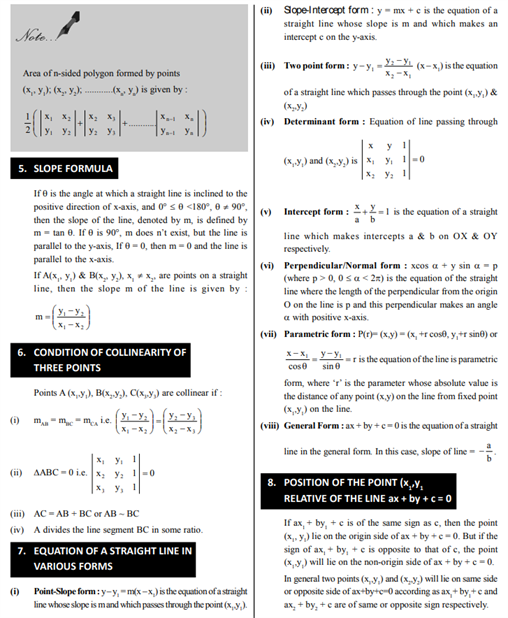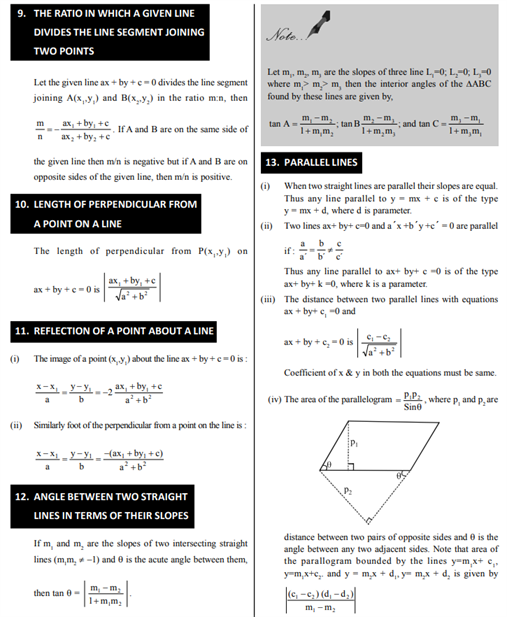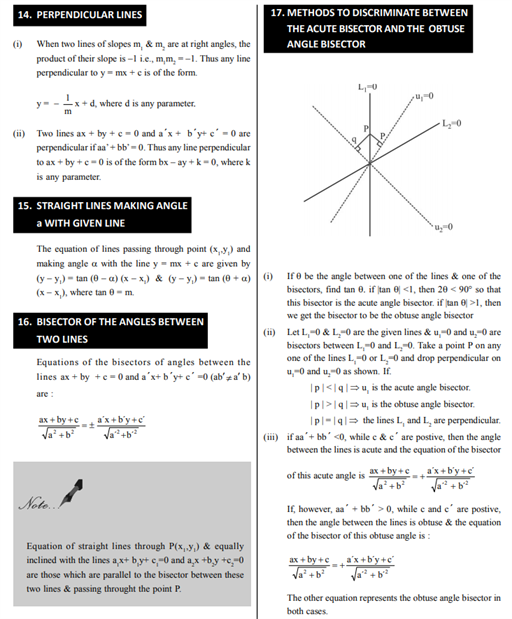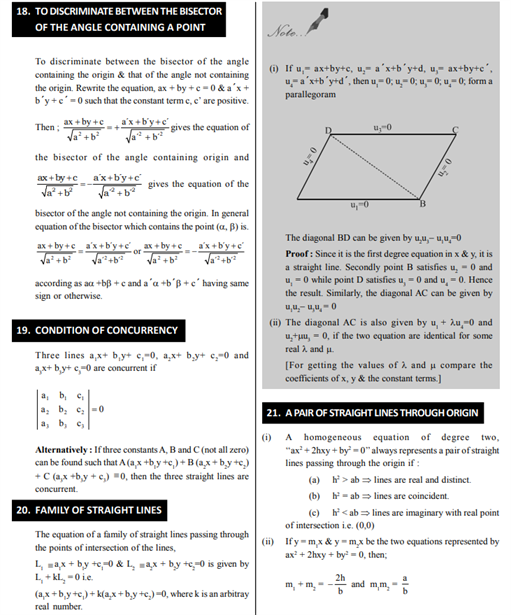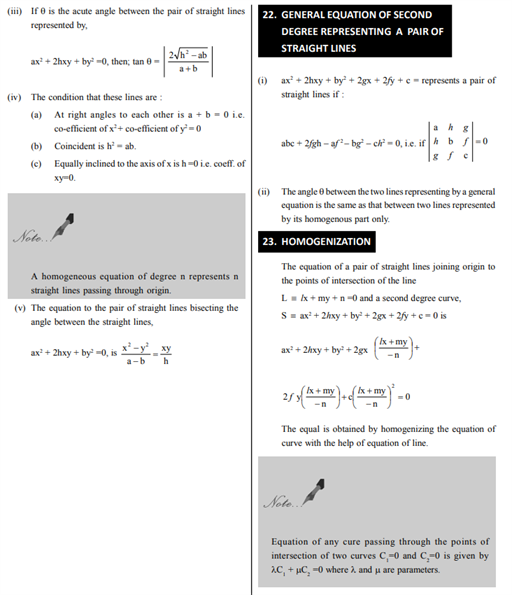# Revision Notes For CBSE Class 11 Maths Chapter 10 Straight Lines

The class 11 maths revision notes chapter 10 Straight Lines bring you the excitement of understanding all the need to know things related to the very important concept in maths which is straight lines

We are aware of the definition of this chapter such as:

• Line can be defined as the shortest distance between two points.
• A line can be defined as a 1-dimensional curve whose direction never change and magnitude is ∞.
• Line can be defined as a cluster of ∞ line segments with their ends in nature.
• Line can be defined as a cluster of ∞ points without changing direction.
• A line can be defined as a linear equation both in rectangular and oblique axes coordinate geometry
• Line is an entity which has ∞ length and 0 thickness.

Students will get all your doubts clarified on important terms such as distance formula, section formula, and slope formula. The most important concept that is the homogenization is explained here in a beautiful manner for their convenience. Students will discover the various conditions of concurrency and along with that, they will also get to know the conditions of perpendicular lines etc.

Check out the class 11 maths revision notes chapter 10 Straight Lines below.